# pkurtosis

Spectral kurtosis from signal or spectrogram

## Syntax

``sk = pkurtosis(x)``
``sk = pkurtosis(x,sampx)``
``sk = pkurtosis(xt)``
``sk = pkurtosis(___,window)``
``sk = pkurtosis(s,sampx,f,window)``
``[sk,fout] = pkurtosis(___)``
``[___,thresh] = pkurtosis(___,'ConfidenceLevel',p) ``
``pkurtosis(___)``

## Description

example

````sk = pkurtosis(x)` returns the spectral kurtosis of vector `x` as the vector `sk`. `pkurtosis` uses normalized frequency (evenly spaced frequency vector spanning [0 π]) to compute the time values. `pkurtosis` computes the spectrogram of `x` using `pspectrum` with default window size (time resolution in samples), and 80% window overlap.```

example

````sk = pkurtosis(x,sampx)` returns the spectral kurtosis of vector `x` sampled at rate or time interval `sampx`. ```
````sk = pkurtosis(xt)` returns the spectral kurtosis of single-variable `timetable` `xt` in the vector `sk`. `xt` must contain increasing finite time samples. ```

example

````sk = pkurtosis(___,window)` returns the spectral kurtosis using the time resolution specified in `window` for the `pspectrum` spectrogram computation. You can use `window` with any of the input arguments in previous syntaxes. ```

example

````sk = pkurtosis(s,sampx,f,window)` returns the spectral kurtosis using the spectrogram or power spectrogram `s`, along with: Sample rate or time, `sampx`, of the original time-series signal that was transformed to produce `s`Spectrogram frequency vector `f`Spectrogram time resolution `window` Use this syntax when you want to customize the options for `pspectrum`, rather than accept the default `pspectrum` options that `pkurtosis` applies. You can specify `sampx` as empty to default to normalized frequency. Although `window` is optional for previous syntaxes, you must supply a value for `window` when using this syntax.```
````[sk,fout] = pkurtosis(___)` returns the spectral kurtosis `sk` along with the frequency vector `fout`. You can use these output arguments with any of the input arguments in previous syntaxes.```

example

````[___,thresh] = pkurtosis(___,'ConfidenceLevel',p) `returns the spectral kurtosis threshold `thresh` using the confidence level `p`. `thresh` represents the range within which the spectral kurtosis indicates a Gaussian stationary signal, at the optional confidence level `p` that you either specify or accept as default. Specifying `p` allows you to tune the sensitivity of the spectral kurtosis `thresh` results to behavior that is non-Gaussian or nonstationary. You can use the `thresh` output argument with any of the input arguments in previous syntaxes. You can also set the confidence level in previous syntaxes, but it has no effect unless you are returning or plotting `thresh`.```
````pkurtosis(___)` plots the spectral kurtosis, along with confidence level and thresholds, without returning any data. You can use this syntax with any of the input arguments in previous syntaxes.```

## Examples

collapse all

Plot the spectral kurtosis of a chirp signal in white noise, and see how the nonstationary non-Gaussian regime can be detected. Explore the effects of changing the confidence level, and of invoking normalized frequency.

Create a chirp signal, add white Gaussian noise, and plot.

```fs = 1000; t = 0:1/fs:10; f1 = 300; f2 = 400; xc = chirp(t,f1,10,f2); x = xc + randn(1,length(t)); plot(t,x) title('Chirp Signal with White Gaussian Noise')```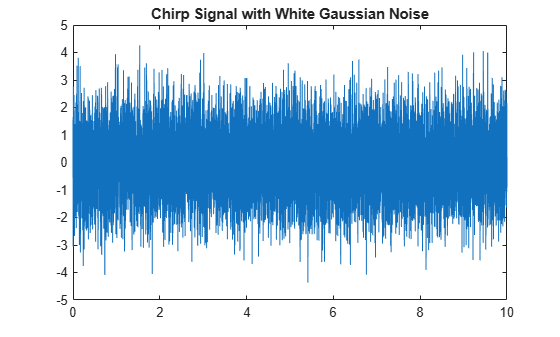Plot the spectral kurtosis of the signal.

```pkurtosis(x,fs) title('Spectral Kurtosis of Chirp Signal with White Gaussian Noise')```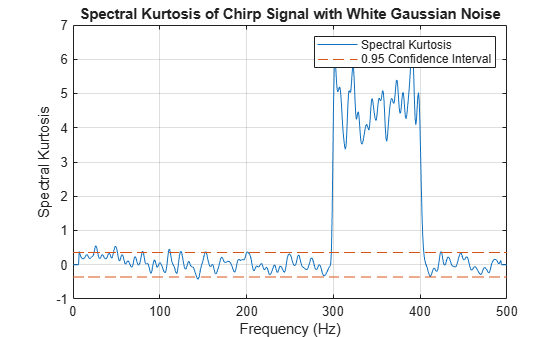The plot shows a clear extended excursion from 300–400 Hz. This excursion corresponds to the signal component which represents the nonstationary chirp. The area between the two horizontal red-dashed lines represents the zone of probable stationary and Gaussian behavior, as defined by the 0.95 confidence interval. Any kurtosis points falling within this zone are likely to be stationary and Gaussian. Outside of the zone, kurtosis points are flagged as nonstationary or non-Gaussian. Below 300 Hz, there are a few additional excursions slightly above the above the zone threshold. These excursions represent false positives, where the signal is stationary and Gaussian, but because of the noise, has exceeded the threshold.

Investigate the impact of the confidence level by changing it from the default 0.95 to 0.85.

```pkurtosis(x,fs,'ConfidenceLevel',0.85) title('Spectral Kurtosis of Chirp Signal with Noise at Confidence Level of 0.85')```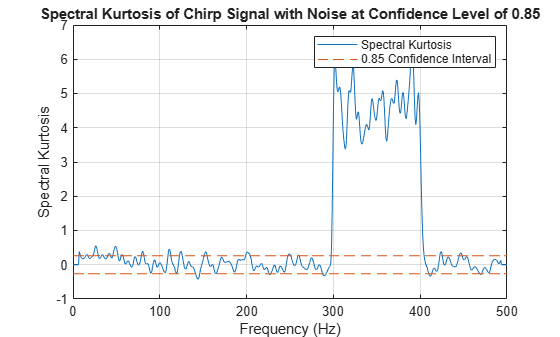The lower confidence level implies more sensitive detection of nonstationary or non-Gaussian frequency components. Reducing the confidence level shrinks the `thresh`-delimited zone. Now the low-level excursions — false alarms — have increased in both number and amount. Setting the confidence level is a balancing act between achieving effective detection and limiting the number of false positives.

You can accurately determine and compare the zone width for the two cases by using the `pkurtosis` form that returns it.

```[sk1,~,thresh95] = pkurtosis(x); [sk2,~,thresh85] = pkurtosis(x,'ConfidenceLevel',0.85); thresh = [thresh95 thresh85]```
```thresh = 1×2 0.3578 0.2628 ```

Plot the spectral kurtosis again, but this time, omit the sample time information so that `pkurtosis` plots normalized frequency.

```pkurtosis(x,'ConfidenceLevel',0.85) title('Spectral Kurtosis using Normalized Frequency')```The frequency axis has changed from Hz to a scale from 0 to π rad/sample.

The `pkurtosis` function uses the default `pspectrum` window size (time resolution). You can specify the window size to use instead. In this example, use the function `kurtogram` to return an optimal window size and use that result for `pkurtosis`.

Create a chirp signal with white Gaussian noise.

```fs = 1000; t = 0:1/fs:10; f1 = 300; f2 = 400; x = chirp(t,f1,10,f2)+randn(1,length(t));```

Plot the spectral kurtosis with the default window size.

```pkurtosis(x,fs) title('Spectral Kurtosis with Default Window Size')```Now compute the optimal window size using `kurtogram`.

`kurtogram(x,fs)`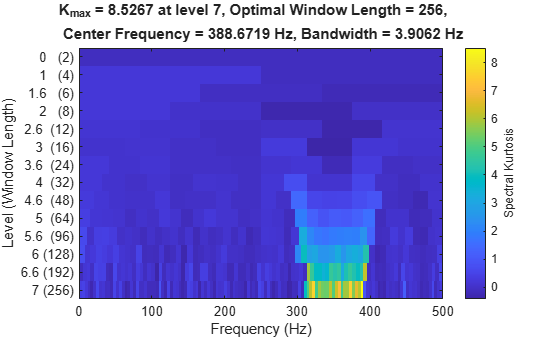The kurtogram plot also illustrates the chirp between 300 and 400 Hz, and shows that the optimum window size is 256. Feed `w0` into `pkurtosis`.

```w0 = 256; pkurtosis(x,fs,w0) title('Spectral Kurtosis with Optimum Window Size of 256')```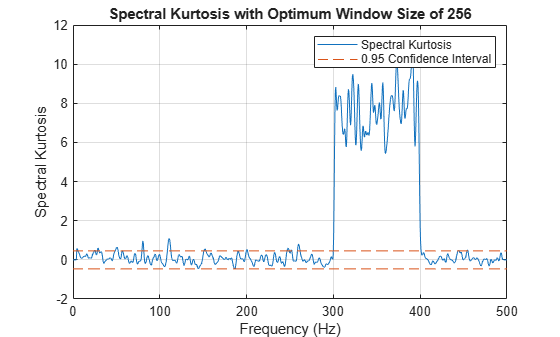The main excursion has higher kurtosis values. The higher values improve the differentiation between stationary and nonstationary components, and enhance your ability to extract the nonstationary component as a feature.

When using signal input data, `pkurtosis` generates a spectrogram by using `pspectrum` with default options. You can also create the spectrogram yourself if you want to customize the options.

Create a chirp signal with white Gaussian noise.

```fs = 1000; t = 0:1/fs:10; f1 = 300; f2 = 400; x = chirp(t,f1,10,f2)+randn(1,length(t));```

Generate a spectrogram that uses your specification for window, overlap, and number of FFT points. Then use that spectrogram in `pkurtosis`.

```window = 256; overlap = round(window*0.8); nfft = 2*window; [s,f,t] = spectrogram(x,window,overlap,nfft,fs); figure pkurtosis(s,fs,f,window)```The magnitude of the excursion is higher, and therefore better differentiated, than with default inputs in previous examples. However, the excursion magnitude here is not as high as it is in the kurtogram-optimized window example.

## Input Arguments

collapse all

Time-series signal from which `pkurtosis` returns the spectral kurtosis, specified as a vector.

Sample rate or sample time, specified as one of the following::

When `sampx` represents a time vector, time samples can be nonuniform, with the `pspectrum` constraint that the median time interval and the mean time interval must obey:

If you specify `sampx` as empty, then `pkurtosis` uses normalized frequency. In other words, it assumes an evenly spaced frequency vector spanning [0 π].

Signal timetable from which `pkurtosis` returns the spectral kurtosis, specified as a `timetable` that contains a single variable with a single column. `xt` must contain increasing, finite row times. If the `timetable` has missing or duplicate time points, you can fix it using the tips in Clean Timetable with Missing, Duplicate, or Nonuniform Times. `xt` can be nonuniformly sampled, with the `pspectrum` constraint that the median time interval and the mean time interval must obey:

Window time resolution to use for the internal `pspectrum` spectrogram computation, specified as a positive scalar in samples. `window` is required for syntaxes that use an existing spectrogram as input, and optional for the rest. You can use the function `kurtogram` to determine the optimal window size to use. `pspectrum` uses 80% overlap by default.

Power spectrogram or spectrum of a signal, specified as a matrix (spectrogram) or a column vector (spectrum).

• If `s` is complex, then `pkurtosis` treats `s` as a short-time Fourier transform (STFT) of the original signal (spectrogram).

• If `s` is real, then `pkurtosis` treats `s` as the square of the absolute values of the STFT of the original signal (power spectrogram). Thus, every element of `s` must be nonnegative.

If you specify `s`, `pkurtosis` uses `s` rather than generate its own spectrogram or power spectrogram. For an example, see Plot Spectral Kurtosis Using a Customized Spectrogram.

Data Types: `single` | `double`
Complex Number Support: Yes

Frequencies for spectrogram or power spectrogram `s` when `s` is supplied explicitly to `pkurtosis`, specified as a vector in hertz. The length of `f` must be equal to the number of rows in `s`.

Confidence level used to determine whether signal is likely to be Gaussian and stationary, specified as a numeric scalar value from 0 to 1. `p` influences the `thresh` range where the spectral kurtosis value indicates a Gaussian and stationary signal. The confidence level therefore provides a detection-sensitivity tuning parameter. Kurtosis values outside of this range indicate, with a probability of (1-`p`), non-Gaussian or nonstationary behavior. For an example, see Plot Spectral Kurtosis of Nonstationary Signal Using Different Confidence Levels.

## Output Arguments

collapse all

Spectral Kurtosis, returned as a double vector. The spectral kurtosis is a statistical quantity that contains low values where data is stationary and Gaussian, and high values where transients occur. One use of the spectral kurtosis is to detect and locate nonstationary or non-Gaussian behavior that could result from faults or degradation. The high-valued kurtosis data reveals such signal components.

Frequencies associated with `sk` values, returned as a vector in hertz.

Spectral kurtosis band size for stationary Gaussian behavior, specified as a numeric scalar representing the thickness of the band centered at the `sk = 0` line, given confidence level `p`. Excursions outside the `thresh`-delimited band indicate possible nonstationary or non-Gaussian behavior. Confidence level `p` directly influences the thickness of the band and the sensitivity of the results. For an example, see Plot Spectral Kurtosis of Nonstationary Signal Using Different Confidence Levels.

collapse all

### Spectral Kurtosis

Spectral kurtosis (SK) is a statistical tool that can indicate and pinpoint nonstationary or non-Gaussian behavior in the frequency domain, by taking:

• Small values at frequencies where stationary Gaussian noise only is present

• High positive values at frequencies where transients occur

This capability makes SK a powerful tool for detecting and extracting signals associated with faults in rotating mechanical systems. On its own, SK can identify features or conditional indicators for fault detection and classification. As preprocessing for other tools such as envelope analysis, SK can supply key inputs such as optimal band , .

The spectral kurtosis, or K(f), of a signal x(t) can be computed based on the short-time Fourier transform (STFT) of the signal, S(t,f):

`$S\left(t,f\right)=\underset{-\infty }{\overset{+\infty }{\int }}x\left(t\right)w\left(t-\tau \right){e}^{-2\pi ft}dt,$`

where w(t) is the window function used in STFT. K(f) is calculated as:

`$K\left(f\right)=\frac{〈{|S\left(t,f\right)|}^{4}〉}{{〈{|S\left(t,f\right)|}^{2}〉}^{2}}-2,f\ne 0,$`

where $〈·〉$ is the time-average operator.

If the signal x(t) contains only stationary Gaussian noise, then K(f) at each frequency f has an asymptotic normal distribution with 0 mean and variance 4/M , where M is the number of elements along the time axis in S(t,f). Hence, a statistical threshold ${s}_{\alpha }$ given a confidence level α is:

`${s}_{\alpha }={\Phi }^{-1}\left(\alpha \right)\frac{2}{\sqrt{M}},$`

where ${\Phi }^{-1}$ is the quantile function of the standard normal distribution.

It is important to note that the STFT window length Nw directly drives frequency resolution, which is fs/Nw, where fs is the sample rate. The window size must be shorter than the spacing between transient impulses, but longer than the individual transient impulses.

 Antoni, J. "The Spectral Kurtosis: A Useful Tool for Characterising Non-Stationary Signals." Mechanical Systems and Signal Processing. Vol. 20, Issue 2, 2006, pp. 282–307.

 Antoni, J., and R. B. Randall. "The Spectral Kurtosis: Application to the Vibratory Surveillance and Diagnostics of Rotating Machines." Mechanical Systems and Signal Processing. Vol. 20, Issue 2, 2006, pp. 308–331.